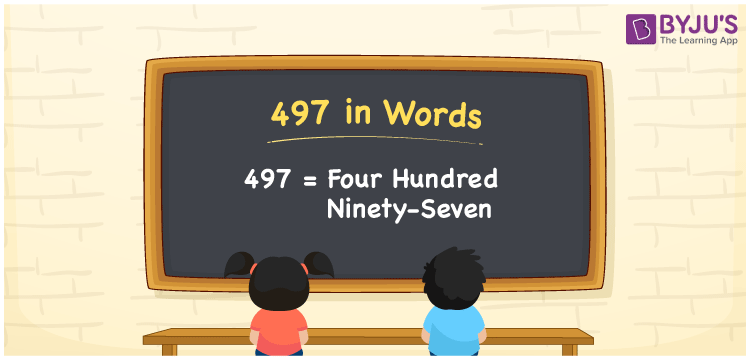# 497 in Words

The number 497 in words is “four hundred ninety-seven”. The numeral 497 contains 3 digits and its place values help us to write the number name. In this article, we will discuss in detail about the procedure of writing the number 497 in words with the help of the place value system.

 497 in Words: Four Hundred Ninety-seven. Four Hundred Ninety-seven in Numerical Form: 497.

## 497 in English Words## How to Write 497 in Words?

The following table presents the place values of the numeral 497.

 Hundreds Tens Ones 4 9 7

The expanded form of 497 is as follows:

= 4 × Hundred + 9 × Ten + 7 × One

= 4 × 100 + 9 × 10 + 7 × 1

= 400 + 90 + 7

= 497

= Four hundred ninety-seven

Hence, 497 in words is four hundred ninety-seven.

497 in words – Four hundred ninety-seven

Is 497 an odd number? – Yes

Is 497 an even number? – No

Is 497 a perfect square number? – No

Is 497 a perfect cube number? – No

Is 497 a prime number? – No

Is 497 a composite number? – Yes

## Frequently Asked Questions on 497 in Words

Q1

### How to write 497 in English words?

497 in words is four hundred ninety-seven.

Q2

### Simplify 400 + 97, and express it in words.

Simplifying 400 + 97, we get 497. Hence, 497 in words is four hundred ninety-seven.

Q3

### Is 497 a composite number?

Yes, 497 is a composite number.